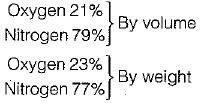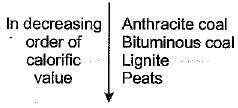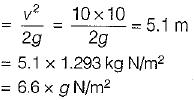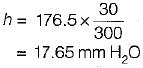Courses

# Test: Fuels & Combustion - 3

## 15 Questions MCQ Test Topicwise Question Bank for Mechanical Engineering | Test: Fuels & Combustion - 3

Description
This mock test of Test: Fuels & Combustion - 3 for Mechanical Engineering helps you for every Mechanical Engineering entrance exam. This contains 15 Multiple Choice Questions for Mechanical Engineering Test: Fuels & Combustion - 3 (mcq) to study with solutions a complete question bank. The solved questions answers in this Test: Fuels & Combustion - 3 quiz give you a good mix of easy questions and tough questions. Mechanical Engineering students definitely take this Test: Fuels & Combustion - 3 exercise for a better result in the exam. You can find other Test: Fuels & Combustion - 3 extra questions, long questions & short questions for Mechanical Engineering on EduRev as well by searching above.
QUESTION: 1

### Efficiency of chimney draught is of the order of

Solution:

The efficiency of a chimney is proportional to the height but even for a very tall chimney, the efficiency will be less than 1% and thus chimney is very inefficient as an instrument for creating draught.

QUESTION: 2

### The percentage O2 by weight and by volume in atmospheric air is

Solution:QUESTION: 3

### For maximum discharge of hot gases through the chimney, the height of hot-gas column producing draught is

Solution:
QUESTION: 4

Calorific value of gaseous fuels can be found be

Solution:

Bomb calorimeter is used for determining the higher calorific value of solid fuels.

QUESTION: 5

Which one of the following coal variety has least calorific value?

Solution:QUESTION: 6

If x1 and x2 be the dryness fractions obtained in separating calorimeter and throttling calorimeter respectively, then the actual dryness friction of steam will be

Solution:

For the combined throttling separating calorimeter, if x1 is the dryness fraction obtained in separating colorimeter and x2 is the dryness fraction obtained in throttling calorimeter then the actual dryness fraction
x = x1x2

QUESTION: 7

In a coupled separating and throttling calorimeter, 0.9. is the dryness fraction of steam in the separating unit and 0.95 is the dryness fraction in the throttling unit. What is the approximate value of the dryness fraction of the steam sample?

Solution:

x = x1x2
x = 0.9 x 0.95
= 0.855

QUESTION: 8

A thermal electric power plant produces 1000 MW of power. If the coal releases 900 x 107 kJ/h of energy, then what is the rate at which heat is rejected from the power plant?

Solution:

Energy released by the coal
= 900 x 107 kJ/hr
= 2500 MW
Heat rejected from the power plant
2500-1000 = 1500 MW

QUESTION: 9

The higher calorific value of fuel occurs when water vapour

Solution:
QUESTION: 10

Forced draught fan delivers air at 10 metres/sec against a draught of 25 mm water across the fuel bed on the grate.
The total draught developed by the force draught fan is

Solution:

Draught across the fuel bed
= 25 mm of water
= g x 25 N/m2
Draught to impart the velocity headTotal draught developed by the force fan
= g(25 + 6.6) = 310 N/m2

QUESTION: 11

Consider the following statements:
1. Induced draught fans require water-cooled bearings.
2. For an induced draught fan installation, the fan size and power requirements are less than those of a forced draught fan installation.
Q. Which of the statements given is/are correct?

Solution:

Induced draught fans are bigger in size as compared to forced draught hence requires more power.

QUESTION: 12

For maximum discharge the draught in mm of H2O is given by (H = height of chimney, T = chimney gas temperature, T1 = atm temperature)

Solution:
QUESTION: 13

For a given height of chimney and its cross-sectional area, the maximum discharge through the chimney is given by: (T1 = atmospheric temp, T = chimney gas temp; m = mass of air/kg fuel)

Solution:
QUESTION: 14

Draught produced by stack 30 meters high for maximum rate of discharge of hot gases through it at 27°C is

Solution:

Draught,QUESTION: 15

Power required by fan in forced draught is.... to that of induced draught

Solution: